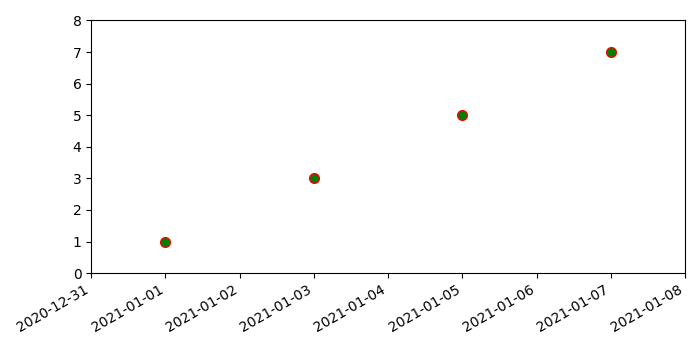# How do I change the range of the X-axis with datetimes in Matplotlib?

To change the range of the X-axis with datetimes in matplotlib, we can take the following steps −

• Create a list of and y, where stores the datetime and stores the number.

• Using subplots method, create a figure and add a set of subplots.

• Plot x and y data points using plots() method, wehere markerface color is green, marker edge color is red, and marker size is 7.

• Since date ticklabels often overlap, so it is useful to rorate them and right-align them using autofmt_xdate() method.

• To change the range of X-axis with datetimes, use set_xlim() with range of datetimes.

• To change the range of Y-axis, use set_ylim() method.

• To display the figure, use show() method.

## Example

import datetime
import matplotlib.pyplot as plt
plt.rcParams["figure.figsize"] = [7.00, 3.50]
plt.rcParams["figure.autolayout"] = True
x = [datetime.date(2021, 1, 1), datetime.date(2021, 1, 3), datetime.date(2021, 1,
5), datetime.date(2021, 1, 7)]
y = [1, 3, 5, 7]
fig, ax = plt.subplots()
ax.plot_date(x, y, markerfacecolor='green', markeredgecolor='red', ms=7)
fig.autofmt_xdate()
ax.set_xlim([datetime.date(2020, 12, 31), datetime.date(2021, 1, 8)])
ax.set_ylim([0, 8])
plt.show()

## Output